MEDIUM
DATA STRUCTURES AND ALGORITHMS

# How to Solve the Partition to K Equal Sum Subsets Problem

ARRAYSSORTINGDYNAMIC PROGRAMMING
Written ByKenny PolyakYibo Gong

## What is the Partition to K Equal Sum Subsets Problem?

The Partition to K Equal Sum Subsets problem involves dividing an array of numbers into k subsets such that the sums of the numbers in each subset are equal. This is a commonly seen problem that tests the application of advanced algorithms like backtracking and dynamic programming.

## 2 Examples of the Partition to K Equal Sum Subsets Interview Question

Given an integer array nums and an integer k, return true if it is possible to divide this array into k non-empty subsets whose sums are all equal.

### Example 1

Input: nums = [4,3,2,3,5,2,1], k = 4
Output: true

Explanation: It is possible to divide `nums` into 4 subsets (5), (1, 4), (2,3), (2,3) with equal sums.

### Example 2

Input: nums = [1,2,3,4], k = 3
Output: false

Constraints

• 1 <= k <= nums.length <= 16
• 1 <= nums[i] <= 104
• The frequency of each element is in the range [1, 4].

## Practice the Partition to K Equal Sum Subsets Problem With Our AI Interviewer

Start AI Interview

## How to Partition to K Equal Sum Subsets: 2 Solutions in Python, Java & JavaScript

To partition the input array into `k` equal subsets, each subset should have a target sum of `total_sum / k`. The naive approach would be to try out all the possibilities by grouping the array elements into `k` subsets with the target sum. We can start by picking the first element in the array, group it with the second element, and see if we can form `k - 1` equal subsets with the remaining elements. If it's not possible, we can remove the second element and try to group the first element with the third element. We can repeat this step until we can reach the `k` equal subsets.

This approach can be modeled with backtracking. Backtracking is a recursive technique that involves breaking a problem into subproblems and solving them to generate a possible decision path k. If the desired solution cannot be reached, we undo whatever decision was made in the previous subproblem and try a different choice.

### Approach 1: Backtracking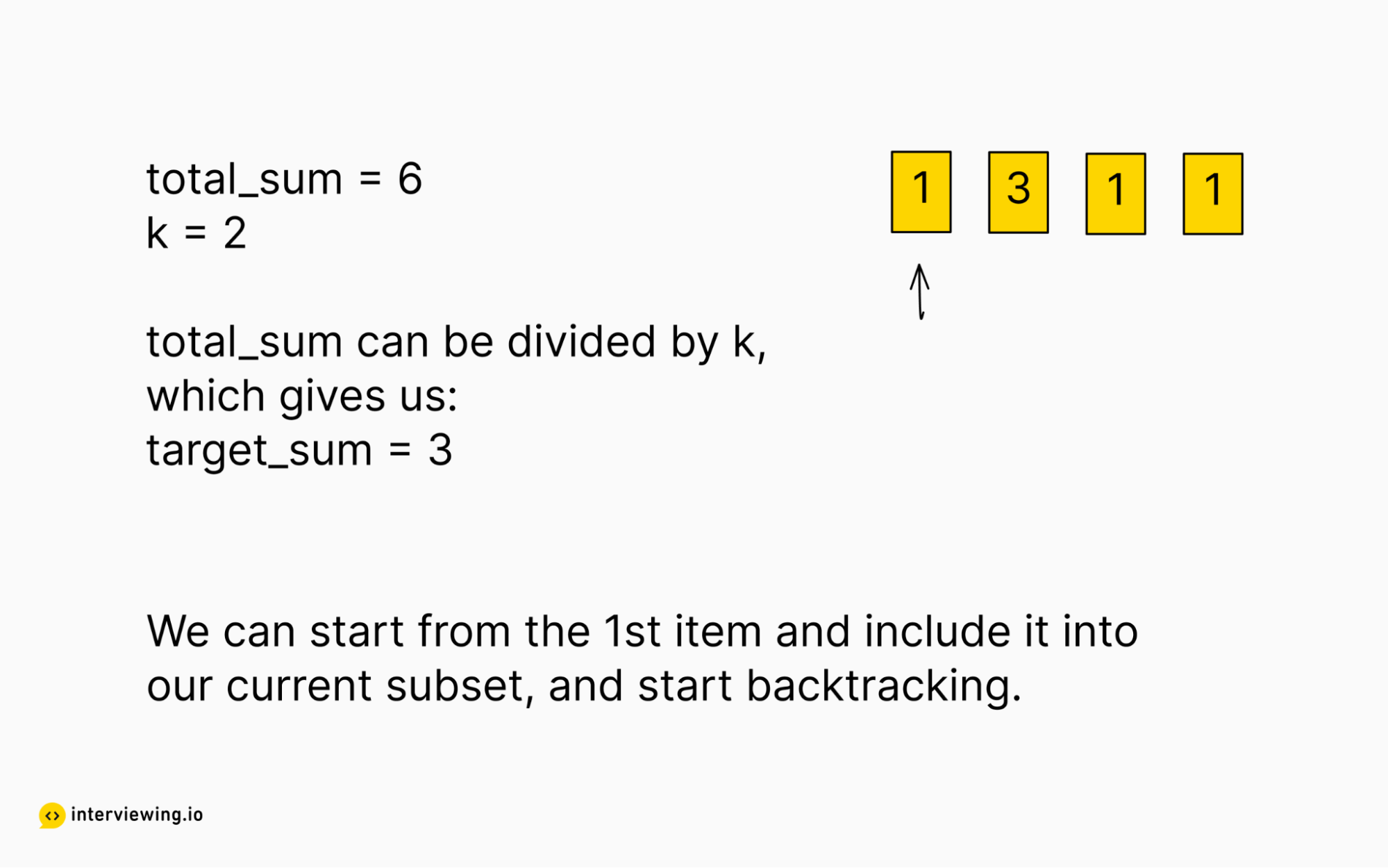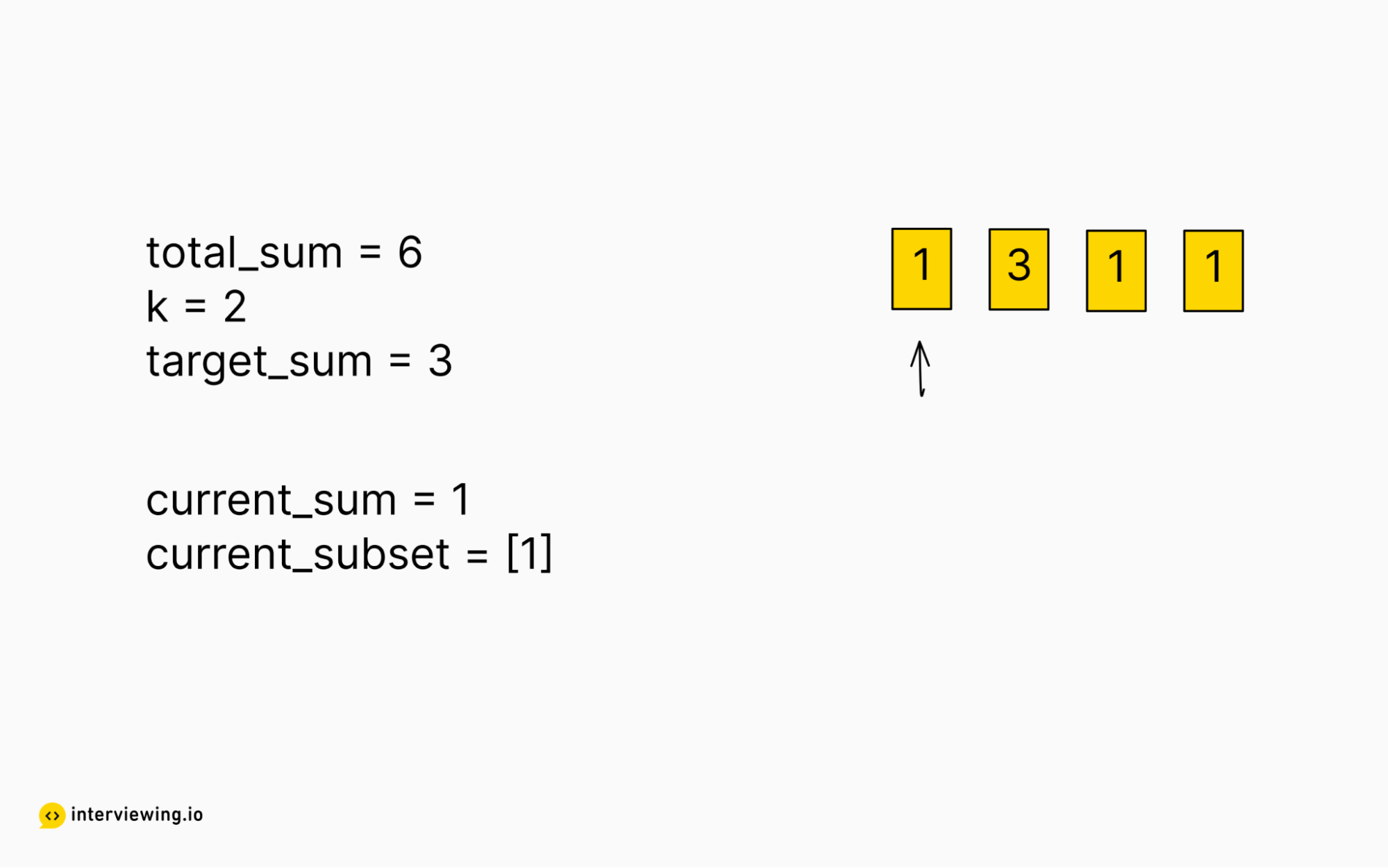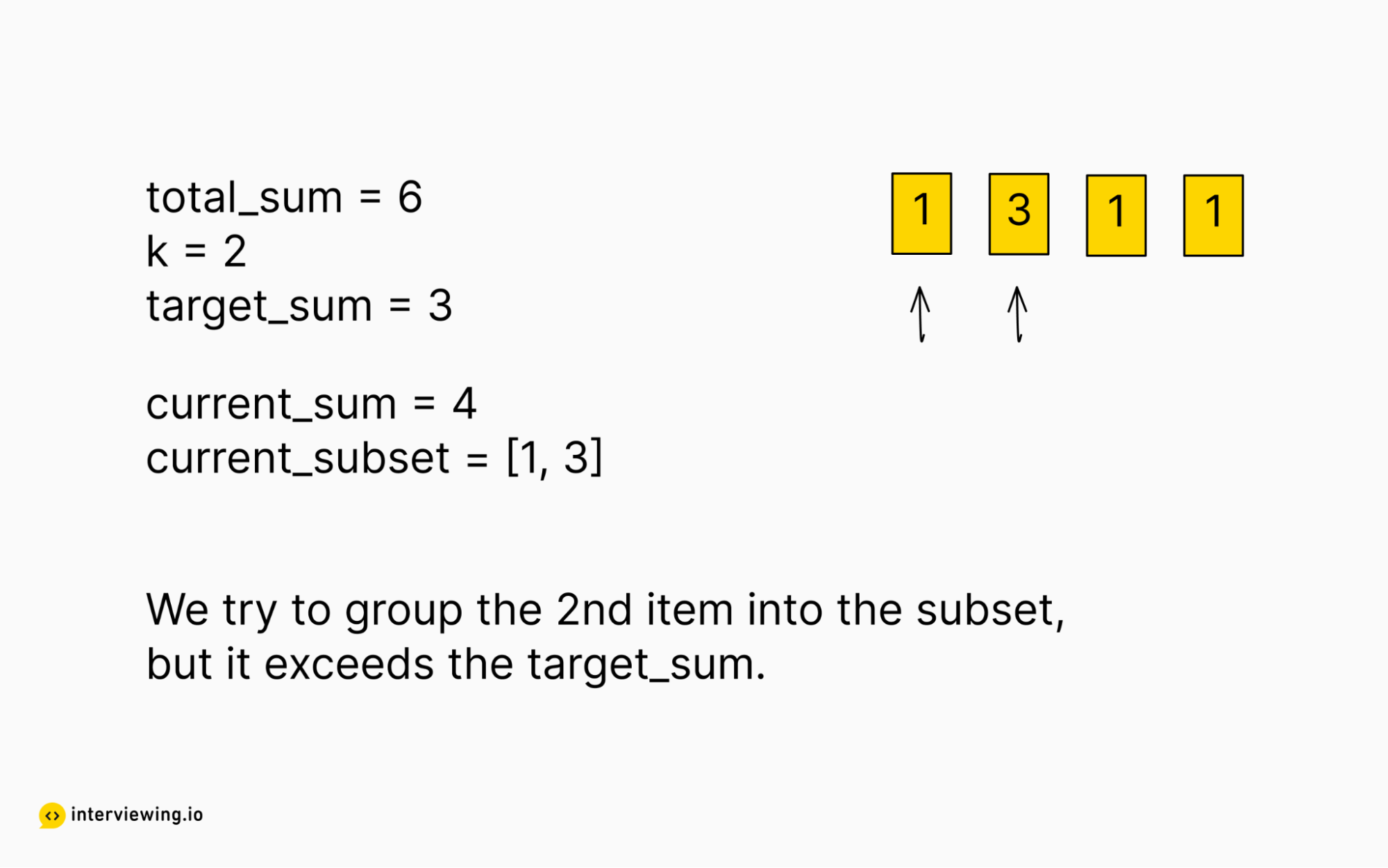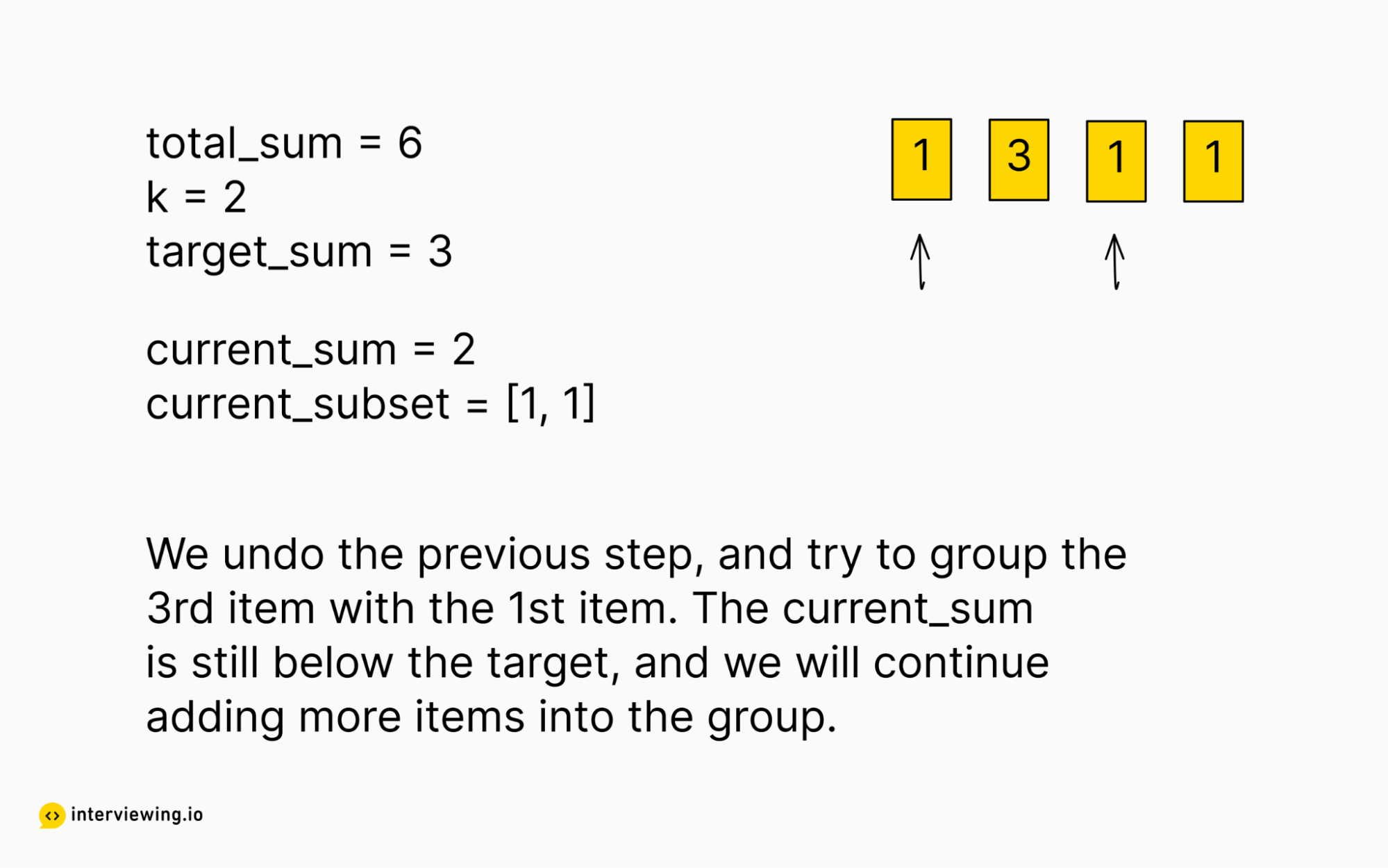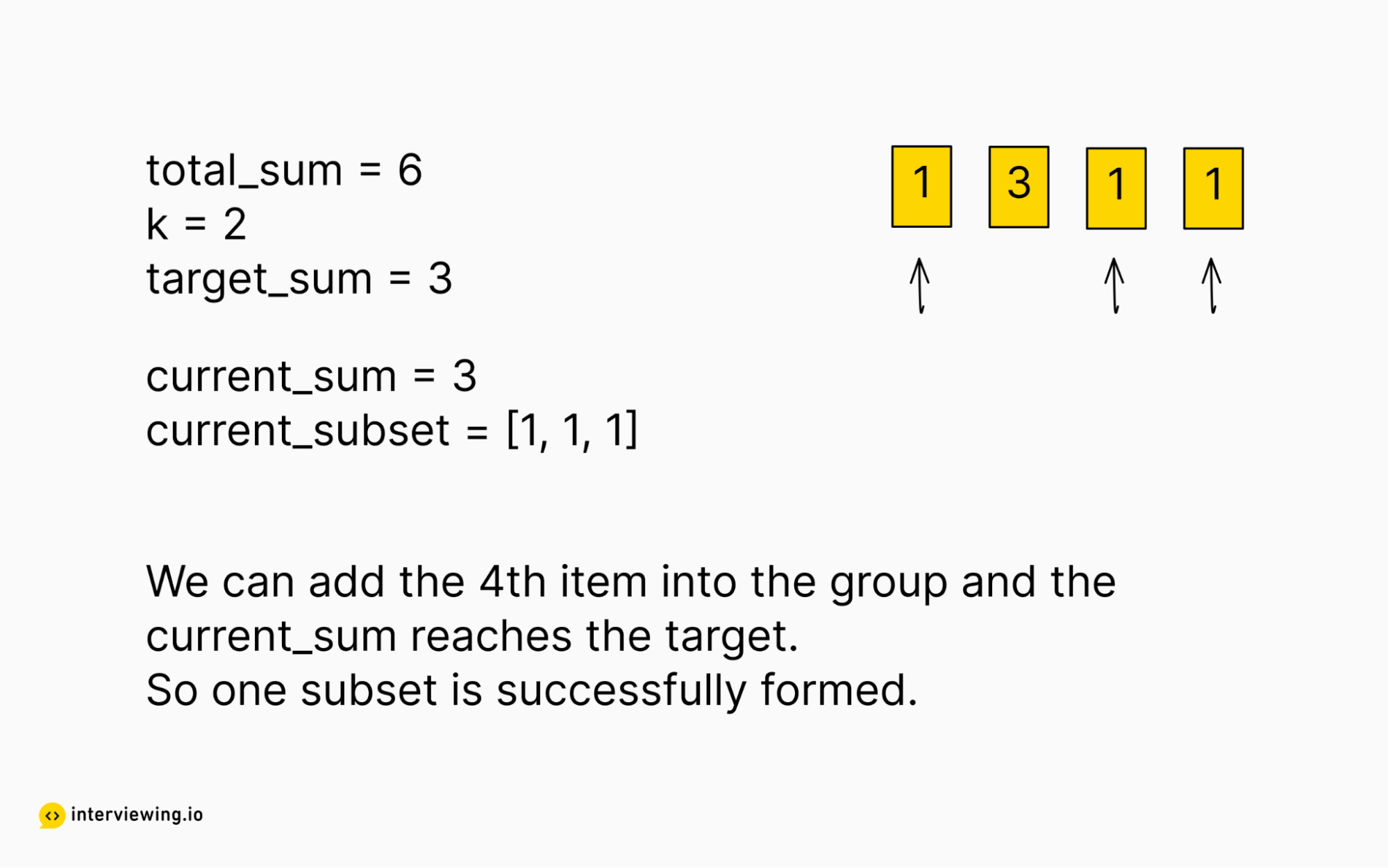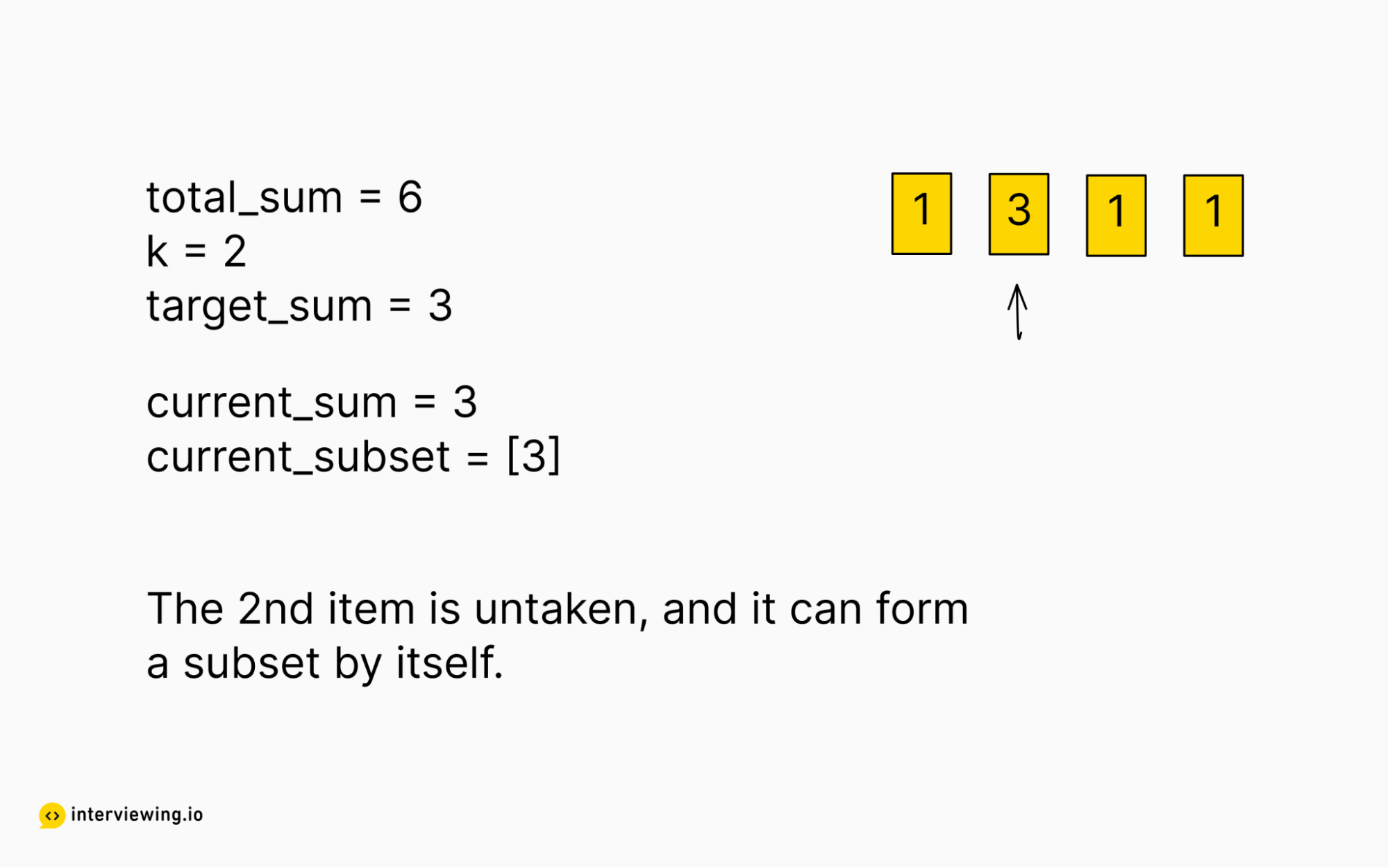To group the array into `k` equal subsets, firstly we need to check if `total_sum` could be divided by `k` or not. If there is a remainder after the division, then it is impossible to group the array into `k` equal subsets; otherwise, we can get the target sum for each subset as `total_sum / k`.

During the recursion, we need to keep track of the following state for each execution context:

1. the number of subsets we have successfully formed
2. the current sum for the current subset
3. the current index of the element in the array we are trying

If we have formed `k - 1` subsets, then we will know for sure that the leftover elements can form the last subset, and the problem is solved. This is the base case where we can return True to stop the current recursion call.

If the current sum is greater than the target sum, then we know for sure this subproblem is not solvable, and we can break out from here. This is the base case where we can return False to stop the current recursion call.

If the current sum is equal to the target sum, then the current subset is complete, and we can start working on a new subset. We can then continue our recursion call with a new current sum as 0.

If the current sum is smaller than the target sum, then we can start trying to add the untaken elements into the current subset to see if we can solve the subproblem. We only need to try out the elements starting with the current index and onwards. There is no need to try out the previous elements as they have already been tried out in previous recursive calls. We will continue our recursion call by incrementing the current sum with the element we pick.

#### Backtracking Solution

``````class Solution:
def canPartitionKSubsets(nums: List[int], k: int) -> bool:
total_sum = sum(nums)

# Return False if the total sum could not be split into K equal subsets
if total_sum % k != 0:
return False

# The target the sum for each subset
target = total_sum // k
# Keep track of the elements that have been taken
taken =  * len(nums)

# input parameters
# - number of subsets we have formed
# - the current sum for the current subset we are working on
# - the index of the element in the array we need to try on
def backtrack(num_completed: int, current_sum: int, index: int) -> bool:
# Return True if we have successfully formed k-1 subsets
# as the last subset will for sure can be formed
if num_completed == k-1:
return True

if current_sum > target:
return False

# Successfully formed one subset, try to form a new one
if current_sum == target:
return backtrack(num_completed + 1, 0, 0)

# Try out each possibility to group into the current subset
for i in range(index, len(nums)):
# try nums[i] if it is not taken
if taken[i] == 0:
taken[i] = 1
# we only need to try out i+1, i+2 elements and onwards
# as the previous elements has already been tried out in previous backtrack loops
if backtrack(num_completed, current_sum + nums[i], i+1):
return True
# undo if nums[i] is not possible
taken[i] = 0

return False

return backtrack(0, 0, 0)``````

#### Time/Space Complexity

• Time Complexity - `O(k * 2^n)` where n is the length of the array and k is the number of subsets
• For each subset, we are trying out all the elements in the array. For each element, either pick it or skip it. So each subset takes 2^n
• For k subset, it will be k * 2^n
• Space Complexity - `O(n)`
• The extra taken array of size n
• The maximum size of the recursive call stack is n, to try out all the elements in the array.

### Approach 2: Optimized backtracking

In the previous solution, we would try to solve a subproblem even if it had already been calculated before. We can improve the time complexity of the previous solution by memorizing the subproblems we have calculated before, so that we can avoid calculating them twice. This will be a tradeoff between time and space.

In our particular case, we can memorize the results for the elements that have already been taken to form a subset. For example, during our recursion, if we encounter a state where the i'th, j'th, and k'th elements are taken, and all the other elements remain untaken, we can memorize the result for this subproblem. Once we encounter the same condition again, we can just read the memorized result without re-calculating it again.

Furthermore, we can break out of a particular recursive call early if we can determine that the subproblem is unsolvable. For a solvable problem, every element should be located into a subset. If, after one backtrack step, we find that we cannot fit the first element into a subset, then we will know for sure this problem is not solvable, and we can return False directly. For example, let us consider the case of `nums = [1, 6, 1, 1, 1], k = 2`, where we need to split the array into 2 subsets with each subset having a sum of 5. We will start recursion on the first element, and try to group it with other elements. After trying out all the possibilities, it turns out that the first element cannot be grouped with others to form a sum of 5. We can simply break out and return False without continuing our recursion.

#### Backtracking With Memorization And Early-Break Solution

``````class Solution:
def canPartitionKSubsets(nums: List[int], k: int) -> bool:
total_sum = sum(nums)

# Return False if the total sum could not be split into K equal subsets
if total_sum % k != 0:
return False

# The target the sum for each subset
target = total_sum // k
# Keep track of the elements that have been taken
taken = ['0'] * len(nums)

# memorize the solutions for the subproblems to avoid duplicate calculations
memo = {}

# input parameters
# - number of subsets we have formed
# - the current sum for the current subset we are working on
# - the index of the element in the array we need to try on
def backtrack(num_completed: int, current_sum: int, index: int) -> bool:
# The current subproblem status
taken_str = ''.join(taken)

# Return True if we have successfully formed k-1 subsets
# as the last subset will for sure can be formed
if num_completed == k-1:
return True

if current_sum > target:
return False

# No need to recalculate if the subproblem has been calculated before
if taken_str in memo:
return memo[taken_str]

# Successfully formed one subset, try to form a new one
if current_sum == target:
memo[taken_str] = backtrack(num_completed + 1, 0, 0)
return memo[taken_str]

# Try out each possibility to group into the current subset
for i in range(index, len(nums)):
# try nums[i] if it is not taken
if taken[i] == '0':
taken[i] = '1'
# we only need to try out i+1, i+2 elements and onwards
# as the previous elements has already been tried out in previous bracktrack loops
if backtrack(num_completed, current_sum + nums[i], i+1):
return True
# undo if nums[i] is not possible
taken[i] = '0'
# Early break. Every element should be grouped into a subset.
# If this element cannot be grouped into a subset, then this problem is not solvable
if taken == ['0'] * len(nums):
break

memo[taken_str] = False
return memo[taken_str]

return backtrack(0, 0, 0)
``````

#### Time/Space Complexity

• Time Complexity - `O(n * 2^n)` where n is the length of the array

• There will be 2^n unique combinations of the taken string
• Every combination takes O(n) time
• Space Complexity - `O(n * 2^n)`

• There will be 2^n unique combinations of the taken string
• Each string takes O(n)

## Watch Mock Interviews Featuring the Partition to K Equal Sum Subsets Question

interviewing.io is a mock interview platform, and people in our community routinely share their interview replays to help others get better. In the video below, you can watch people solve Partition to K Equal Sum Subsets. The interview is in C++. The interviewer is an anonymous senior engineer from FAANG.
FAANG InterviewC++ Interview
Advance this person to the next round?Technical Skills:
4/4
Problem Solving Ability:
4/4
Communication Ability:
3/4